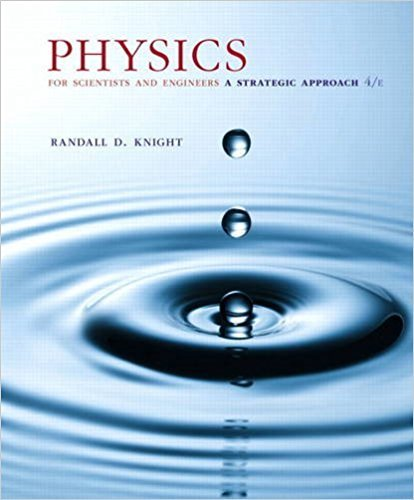×
×

# Charges q1 = -4Q and q2 = +2Q are located at x = -a and xISBN: 9780134081496 191

## Solution for problem 24.59 Chapter 24

Physics for Scientists and Engineers: A Strategic Approach, Standard Edition (Chs 1-36) | 4th Edition

• Textbook Solutions
• 2901 Step-by-step solutions solved by professors and subject experts
• Get 24/7 help from StudySoup virtual teaching assistantsPhysics for Scientists and Engineers: A Strategic Approach, Standard Edition (Chs 1-36) | 4th Edition

4 5 1 395 Reviews
13
1
Problem 24.59

Charges q1 = -4Q and q2 = +2Q are located at x = -a and x = +a, respectively. What is the net electric flux through a sphere of radius 2a centered (a) at the origin and (b) at x = 2a?

Step-by-Step Solution:
Step 1 of 3
Step 2 of 3

Step 3 of 3

##### ISBN: 9780134081496

Physics for Scientists and Engineers: A Strategic Approach, Standard Edition (Chs 1-36) was written by and is associated to the ISBN: 9780134081496. The answer to “Charges q1 = -4Q and q2 = +2Q are located at x = -a and x = +a, respectively. What is the net electric flux through a sphere of radius 2a centered (a) at the origin and (b) at x = 2a?” is broken down into a number of easy to follow steps, and 42 words. This full solution covers the following key subjects: . This expansive textbook survival guide covers 42 chapters, and 4463 solutions. Since the solution to 24.59 from 24 chapter was answered, more than 227 students have viewed the full step-by-step answer. This textbook survival guide was created for the textbook: Physics for Scientists and Engineers: A Strategic Approach, Standard Edition (Chs 1-36), edition: 4. The full step-by-step solution to problem: 24.59 from chapter: 24 was answered by , our top Physics solution expert on 12/28/17, 08:06PM.

Unlock Textbook Solution